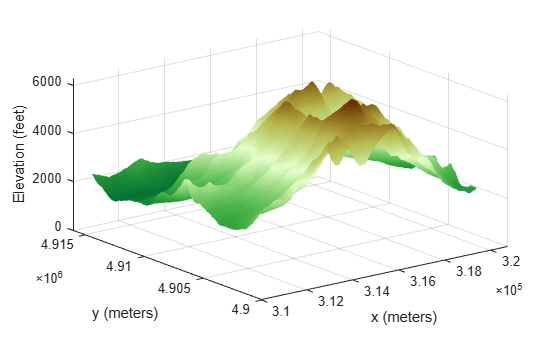worldGrid

World coordinates of raster elements

Description

example

[X,Y] = worldGrid(R) returns the world coordinates of raster elements as the 2-D arrays X and Y. The coordinates of raster element (i,j) are (X(i,j),Y(i,j)).

example

[X,Y] = worldGrid(R,gridOption), where gridOption is 'gridvectors', returns X and Y as row vectors. The coordinates of raster element (i,j) are (X(j),Y(i)). The default for gridOption is 'fullgrid', which returns X and Y as 2-D arrays.

Examples

collapse all

Import elevation data, find the coordinates of the imported data, then display the data as a surface.

First import elevation data  for an area around Mount Washington as an array and a map cells reference object. Prepare the data for plotting by replacing missing data values with 0. Then, find the coordinates of each element in the array.

info = georasterinfo('MtWashington-ft.grd');
Z = standardizeMissing(Z,info.MissingDataIndicator);

[X,Y] = worldGrid(R);

Display the elevation data as a surface using an appropriate colormap. Set the EdgeColor property to 'none' to remove the mesh from the surface.

surf(X,Y,Z,'EdgeColor','none')
demcmap(Z)
xlabel('x (meters)')
ylabel('y (meters)')
zlabel('Elevation (feet)')The elevation data used in this example is courtesy of the U.S. Geological Survey.

Create a map cells reference object for a 3-by-4 raster with x values in the range [7000, 7400] meters and y values in the range [2700, 3300] meters. Get the coordinates of the raster elements and return them as row vectors.

R = maprefcells([7000 7400],[2700 3300],[3 4]);
[X,Y] = worldGrid(R,'gridvectors')
X = 1×4

7050        7150        7250        7350

Y = 1×3

2800        3000        3200

If you do not specify the second argument as 'gridvectors', then the worldGrid function returns 2-D arrays by default.

[xFull,yFull] = worldGrid(R)
xFull = 3×4

7050        7150        7250        7350
7050        7150        7250        7350
7050        7150        7250        7350

yFull = 3×4

2800        2800        2800        2800
3000        3000        3000        3000
3200        3200        3200        3200

Input Arguments

collapse all

Spatial reference, specified as a MapCellsReference or MapPostingsReference object.

If R is a MapCellsReference object, then X and Y are cell centers. If R is a MapPostingsReference object, then X and Y are posting points.

Grid option, specified as one of these values:

• 'fullgrid' — Return X and Y as 2-D arrays, where each row of X is identical and each column of Y is identical. This is the default behavior.

• 'gridvectors' — Return X and Y as row vectors. Use this option when you want to reduce memory usage and when 2-D arrays are unnecessary, such as when plotting large data sets with the surf function. You can specify gridOption as 'gridvectors' only when the TransformationType property of R has a value of 'rectilinear'.

This table shows the difference between 'fullgrid' and 'gridvectors'.

'fullgrid''gridvectors'
R = maprefcells([7000 7400],[2700 3300],[3 4]);
[X,Y] = worldGrid(R)
X =

7050        7150        7250        7350
7050        7150        7250        7350
7050        7150        7250        7350

Y =

2800        2800        2800        2800
3000        3000        3000        3000
3200        3200        3200        3200
R = maprefcells([7000 7400],[2700 3300],[3 4]);
[X,Y] = worldGrid(R,'gridvectors')
X =

7050        7150        7250        7350

Y =

2800        3000        3200

Data Types: char | string

Output Arguments

collapse all

x-coordinates, returned as a 2-D array or a row vector. By default, X is a 2-D array. To return X as a row vector, specify gridOption as 'gridvectors'.

By default, and when gridOption is 'fullgrid', the sizes of X and Y each equal the RasterSize property of R. When gridOption is 'gridvectors', the lengths of X and Y equal the second and first elements of the RasterSize property of R, respectively.

y-coordinates, returned as a 2-D array or a row vector. By default, Y is a 2-D array. To return Y as a row vector, specify gridOption as 'gridvectors'.

By default, and when gridOption is 'fullgrid', the sizes of X and Y each equal the RasterSize property of R. When gridOption is 'gridvectors', the lengths of X and Y equal the second and first elements of the RasterSize property of R, respectively.

Objects

Introduced in R2021a

Mapping Toolbox Documentation